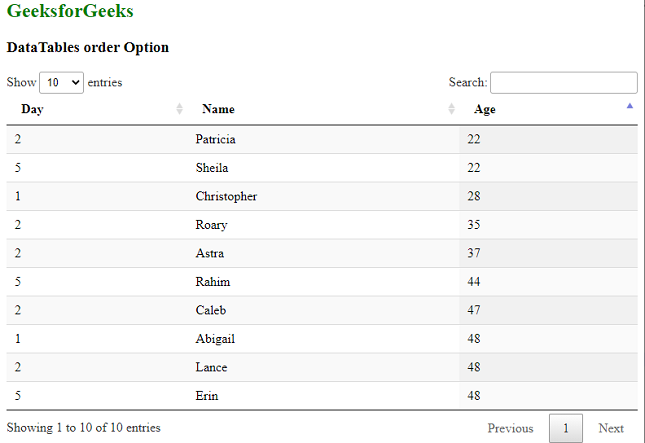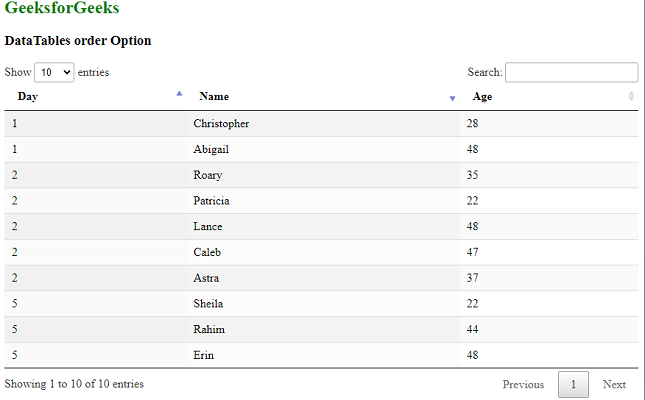# DataTables order Option

• Last Updated : 13 Jul, 2021

DataTables is a jQuery plugin that can be used for adding interactive and advanced controls to HTML tables for the webpage. This also allows the data in the table to be searched, sorted, and filtered according to the needs of the user. The DataTables also exposes a powerful API that can be further used to modify how the data is displayed.

The order option is used to specify the rows of the DataTable that have to be ordered and their direction. It accepts a two-dimensional array that allows the ordering to be specified in multiple columns at once. The column defined first would be given more priority in sorting. The default value is [[0, ‘asc’]], which means that only the first column is sorted in ascending order.

Syntax:

`{ order: value }`

Parameters: This option has a single value as mentioned above and described below.

• value: This is a multi-dimension array value that specifies the rows and direction that the columns are to be sorted. The first value in each of the arrays is the column number to be ordered, which starts from ‘0’ and the second one is its direction, which can be the values ‘asc‘ or ‘desc‘.

Example 1: In this example, the 3rd column is sorted in ascending order.

## HTML

 `<``html``>``<``head``>``  ````  ``<``script` `type``=``"text/javascript"` `          ``src``=``"https://code.jquery.com/jquery-3.5.1.js"``>``  ```` ` `  ````  ``<``link` `rel``=``"stylesheet"``        ``href``=``"https://cdn.datatables.net/1.10.23/css/jquery.dataTables.min.css"``>`` ` `  ````  ``<``script` `src``=``"https://cdn.datatables.net/1.10.23/js/jquery.dataTables.min.js"``>``  ``````<``body``>``  ``<``h1` `style``=``"color: green;"``>``    ``GeeksForGeeks``  ````  ``<``h3``>DataTables order Option`` ` `  ````  ``<``table` `id``=``"tableID"` `class``=``"display nowrap"``>``    ``<``thead``>``      ``<``tr``>``        ``<``th``>Day``        ``<``th``>Name``        ``<``th``>Age``      ````    ````    ``<``tbody``>``      ``<``tr``>``        ``<``td``>2``        ``<``td``>Patricia``        ``<``td``>22``      ````      ``<``tr``>``        ``<``td``>2``        ``<``td``>Caleb``        ``<``td``>47``      ````      ``<``tr``>``        ``<``td``>1``        ``<``td``>Abigail``        ``<``td``>48``      ````      ``<``tr``>``        ``<``td``>5``        ``<``td``>Rahim``        ``<``td``>44``      ````      ``<``tr``>``        ``<``td``>5``        ``<``td``>Sheila``        ``<``td``>22``      ````      ``<``tr``>``        ``<``td``>2``        ``<``td``>Lance``        ``<``td``>48``      ````      ``<``tr``>``        ``<``td``>5``        ``<``td``>Erin``        ``<``td``>48``      ````      ``<``tr``>``        ``<``td``>1``        ``<``td``>Christopher``        ``<``td``>28``      ````      ``<``tr``>``        ``<``td``>2``        ``<``td``>Roary``        ``<``td``>35``      ````      ``<``tr``>``        ``<``td``>2``        ``<``td``>Astra``        ``<``td``>37``      ````    ````  ````  ``<``script``>`` ` `    ``// Initialize the DataTable``    ``\$(document).ready(function () {``      ``\$('#tableID').DataTable({`` ` `        ``// Set the 3rd column of the``        ``// DataTable to ascending order``        ``order: [[2, 'asc']]``      ``});``    ``}); ``  ```````

Output:Example 2: In this example, the 1st column is sorted in ascending order, and then the 2nd column is sorted in descending order.

## HTML

 `<``html``>``<``head``>``  ````  ``<``script` `type``=``"text/javascript"` `          ``src``=``"https://code.jquery.com/jquery-3.5.1.js"``>``  ```` ` `  ````  ``<``link` `rel``=``"stylesheet"``        ``href``=``"https://cdn.datatables.net/1.10.23/css/jquery.dataTables.min.css"``>`` ` `  ````  ``<``script` `src``=``"https://cdn.datatables.net/1.10.23/js/jquery.dataTables.min.js"``>``  ````  ``<``style``>``     ``th``      ``{``       ``text-align:left; ``      ``}``  ``````<``body``>``  ``<``h2` `style``=``"color:green;"``>``    ``GeeksForGeeks``  ````  ``<``h3``>DataTables order Option`` ` `  ````  ``<``table` `id``=``"tableID"` `class``=``"display nowrap"``>``    ``<``thead``>``      ``<``tr``>``        ``<``th``>Day``        ``<``th``>Name``        ``<``th``>Age``      ````    ````    ``<``tbody``>``      ``<``tr``>``        ``<``td``>2``        ``<``td``>Patricia``        ``<``td``>22``      ````      ``<``tr``>``        ``<``td``>2``        ``<``td``>Caleb``        ``<``td``>47``      ````      ``<``tr``>``        ``<``td``>1``        ``<``td``>Abigail``        ``<``td``>48``      ````      ``<``tr``>``        ``<``td``>5``        ``<``td``>Rahim``        ``<``td``>44``      ````      ``<``tr``>``        ``<``td``>5``        ``<``td``>Sheila``        ``<``td``>22``      ````      ``<``tr``>``        ``<``td``>2``        ``<``td``>Lance``        ``<``td``>48``      ````      ``<``tr``>``        ``<``td``>5``        ``<``td``>Erin``        ``<``td``>48``      ````      ``<``tr``>``        ``<``td``>1``        ``<``td``>Christopher``        ``<``td``>28``      ````      ``<``tr``>``        ``<``td``>2``        ``<``td``>Roary``        ``<``td``>35``      ````      ``<``tr``>``        ``<``td``>2``        ``<``td``>Astra``        ``<``td``>37``      ````    ````  ````  ``<``script``>`` ` `    ``// Initialize the DataTable``    ``\$(document).ready(function () {``      ``\$('#tableID').DataTable({`` ` `        ``// Set the 1st column of the``        ``// DataTable to ascending order``        ``// and the 2nd to descending order``        ``order: [[0, 'asc'], [1, 'desc']]``      ``});``    ``}); ``  ```````

Output:ascending order of 1st and descending order of 2nd column